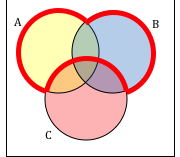## Venn Diagrams

To visualize the interaction of sets, John Venn in 1880 thought to use overlapping circles, building on a similar idea used by Leonhard Euler in the 18th century. These illustrations now called Venn Diagrams.

### Venn Diagram

A Venn diagram represents each set by a circle, usually drawn inside of a containing box representing the universal set. Overlapping areas indicate elements common to both sets.

Basic Venn diagrams can illustrate the interaction of two or three sets.

### Example 9

Create Venn diagrams to illustrate A B, A B, and Ac B

A B contains all elements in either set.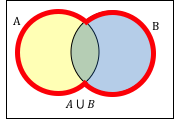A B contains only those elements in both sets – in the overlap of the circles.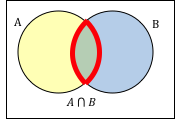Ac will contain all elements not in the set A. Ac B will contain the elements in set B that are not in set A.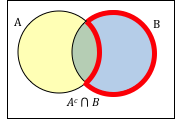### Example 10

Use a Venn diagram to illustrate (H F)c W

We’ll start by identifying everything in the set H F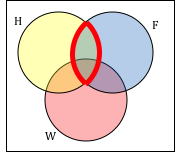Now, (H F)c W will contain everything not in the set identified above that is also in set W.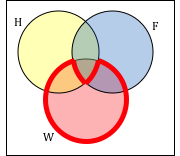### Example 11

Create an expression to represent the outlined part of the Venn diagram shown.

The elements in the outlined set are in sets H and F, but are not in set W. So we could represent this set as H F Wc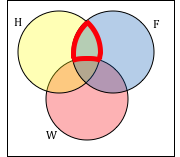### Try it Now 3

Create an expression to represent the outlined portion of the Venn diagram shown